Please visit, subscribe and share 10 Minutes Lectures in Computer Science

## Bigram Model - Probability Calculation - Example Problem

Solved Example:

Let us solve a small example to better understand the Bigram model. For this we need a corpus and the test data. Let us assume that the following is a small corpus;

Training corpus:
<s> I am from Vellore </s>
<s> I am a teacher </s>
<s> students are good and are from various cities</s>
<s> students from Vellore do engineering</s>

Test data:
<s> students are from Vellore </s>

Let us find the Bigram probability of the given test sentence. I explained the solution in two methods, just for the sake of understanding. the second method is the formal way of calculating the bigram probability of a sequence of words.

Method 1
As per the Bigram model, the test sentence can be expanded as follows to estimate the bigram probability;
P(<s> students are from Vellore </s>)
= P(students | <s>) * P(are | students) * P(from | are)
* P(Vellore | from) *    P(</s> | Vellore)

To estimate bigram probabilities, we can use the following equation;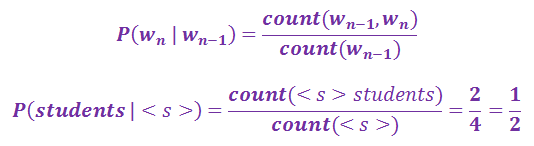[Hint – count of sentence start (<s>) = 4, count of string <s> students = 1]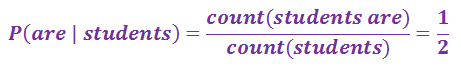[Hint – count of word students = 2, count of string students are = 1]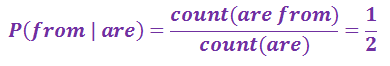[Hint – count of word are = 2, count of string are from = 1]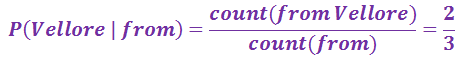[Hint – count of word from = 3, count of string from Vellore = 2]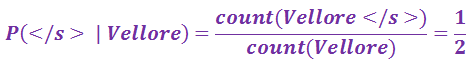[Hint – count of word Vellore = 2, count of string Vellore </s> = 1]
P(<s> students are from Vellore </s>)
= P(students | <s>) * P(are | students) * P(from | are)
* P(Vellore | from) * P(</s> | Vellore)
= 1/4 * 1/2 * 1/2 * 2/3 * 1/2 = 0.0208

Method 2
Formal way of estimating the bigram probability of a word sequence:
The bigram probabilities of the test sentence can be calculated by constructing Unigram and bigram probability count matrices and bigram probability matrix as follows;
Unigram count matrix
 students are from Vellore 4 2 2 3 2

Bigram count matrix
 wn students are from Vellore wn-1 1 0 0 0 0 students 0 1 1 0 0 are 0 0 1 0 0 from 0 0 0 2 0 Vellore 0 0 0 0 1

Bigram probability matrix (normalized by unigram counts)
 wn students are from Vellore wn-1 1/4 0/4 0/4 0/4 0/4 students 0/2 1/2 1/2 0/2 0/2 are 0/2 0/2 1/2 0/2 0/2 from 0/3 0/3 0/3 2/3 0/3 Vellore 0/2 0/2 0/2 0/2 1/2

P(<s> students are from Vellore </s>)
= P(students | <s>) * P(are | students) * P(from | are)
* P(Vellore | from) * P(</s> | Vellore)
= 1/4 * 1/2 * 1/2 * 2/3 * 1/2 = 0.0208

The probability of the test sentence as per the bigram model is 0.0208.

----------------------------------------------------------------------------------------------------------

# How to calculate probability of a sentence as per bigram statistical language model

#### Bigram model solved exercises

1.you have made a mistake in the first question it must be 2/4

1.thanks. It should be 2/4.

2.nice explanation.

## Featured Content

### Multiple choice questions in Natural Language Processing Home

MCQ in Natural Language Processing, Quiz questions with answers in NLP, Top interview questions in NLP with answers Multiple Choice Que...

data recovery